Know more about the Repeaters Batch Know more about the Repeaters Batch

# JEE Advanced Question Paper 2021 Chemistry Paper 1

JEE Advanced 2021 Chemistry question paper for Paper 1 is available here. Along with the questions, we have also provided solutions to each problem given in the question paper. The solutions have been prepared by our expert faculty in an easy-to-understand manner. This will further help students to not only find the correct answers but also figure out how the outcome was achieved. Going through the JEE Advanced 2021 Chemistry questions and solutions will allow students to further develop better problem-solving and time management skills. Additionally, they will be able to study more productively for the upcoming entrance exam. Students can access the JEE Advanced 2021 Paper 1 Chemistry question paper and solution PDFs below. We have also provided paper analysis videos which students can watch and learn more about the question types, weightage of marks, important concepts and more.

### JEE Advanced 2021 Paper 1 Chemistry Question Paper

Question 1. The major product formed in the following reaction is: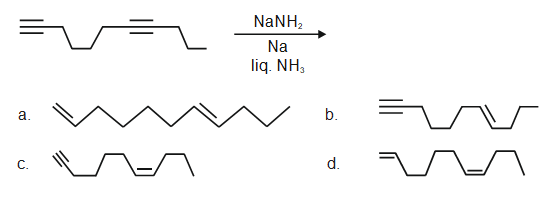Solution:

It is a case of Birch reduction. Alkynes on reaction with alkali metal in liq. NH3 gives trans-alkene. But terminal alkynes do not get reduced.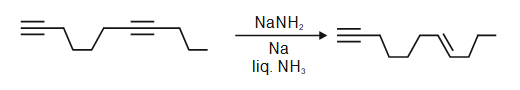Question 2. Among the following, the conformation that corresponds to the most stable conformation of meso-butane-2,3-diol is;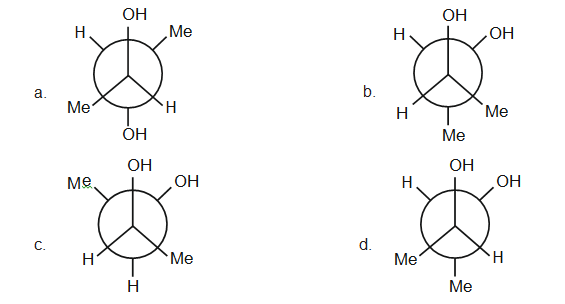Solution:

Meso compounds have a plane of symmetry. In case of butan-2, 3-diol, gauche form is the most stable due to intramolecular H-bonding.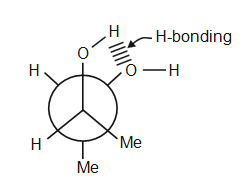Question 3. For the given close-packed structure of a salt made of cation X and anion Y shown below (ions of only one face are shown for clarity), the packing fraction is approximately (packing fraction = packing efficiency / 100)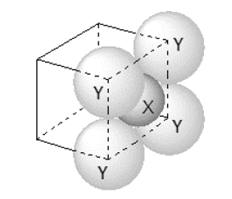1. a. 0.74
2. b. 0.63
3. c. 0.52
4. d. 0.48

Solution:

a = edge length of unit cell

2ry = a

2 (rx - ry) = √2a

2rx + a = √2a

2rx = a (√2a - 1)

rx = 0.207 a

Packing fraction = 3 × vol. of x + vol. of y / vol. of unit cell

$$\frac{3\times \frac{4}{3}\times \pi r_{x}^{3}+\frac{4}{3}+\pi \times r_{y}^{3}}{a^{3}}$$

$$\frac{4\times \pi \times (0.207a)^{3}+\frac{4}{3}\times\pi \times(0.5a)^{3}}{a^{3}}$$

Question 4. The calculated spin only magnetic moments of [Cr(NH3)6]3+ and [CuF6]3– in BM, respectively, are (Atomic numbers of Cr and Cu are 24 and 29, respectively).

1. a. 3.87 and 2.84
2. b. 4.90 and 1.73
3. c. 3.87 and 1.73
4. d. 4.90 and 2.84

Solution:

[Cr(NH3)6]3+ = Cr3+

Cr3+ = 3d3 4s0

It has 3 unpaired electrons

μ = n √(n+2) BM

μ = 3 √(3+2) BM

μ = 3.87 BM

[CuF6]3- = Cu+3

Cu+3 = 3d8 4s0

It has 2 unpaired electrons

μ = 2 √(2+2) BM

= 2.84 BM

Question Stem for Question 5 and 6:

For the following reaction scheme, percentage yields are given along the arrow: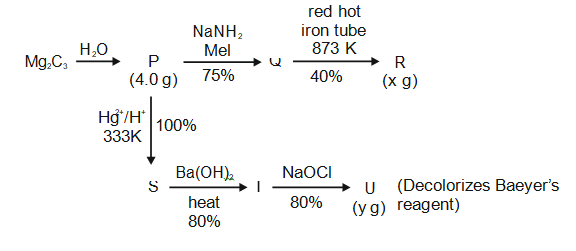x g and y g are the masses of R and U, respectively. (Use: Molar mass (in g mol–1) of H, C and O as 1, 12 and 16, respectively)

Question 5. The value of x is______.

Solution:

Question 6. The value of y is ______.

Solution:

Solution for both Questions 5 and 6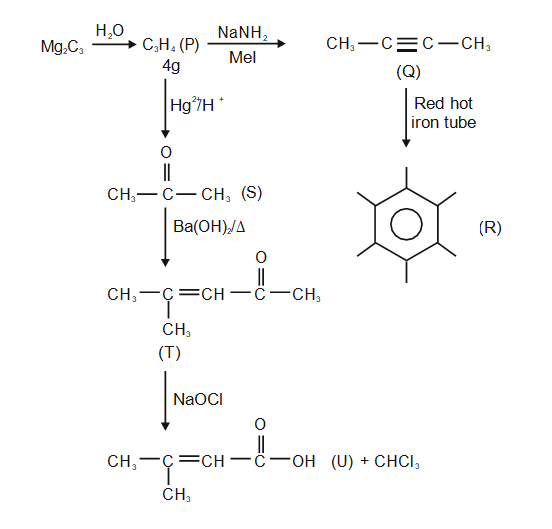4 g of C3H4 = 0.1 mol

From 0.1 mol of P, 0.01 mol of R will be produced

⇒ 1.62 g of R is produced

From 0.1 mol of P, 0.032 mol of U is produced

= 3.2 g of U is produced

Question statement for Questions 7 and 8.

For the reaction, X(s) ⇌ Y(s) + Z(g), the plot of

$$In\frac{p_{z}}{p^{\varnothing}}$$
Versus 104 / T is given below (in solid line), where pz is the pressure (in bar) of the gas Z at temperature T and
$$p^{\varnothing}$$
= 1 bar.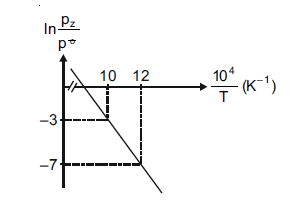(Given,

$$\frac{d(In\:K)}{d\left (\frac{1}{T} \right)} = -\frac{\Delta H^{\varnothing }}{R}$$
, where the equilibrium constant
$$K = \frac{p_{z}}{p^{\varnothing}}$$
and the gas constant, R = 8.314 J K–1 mol–1)

Question 7. The value of standard enthalpy,

$$ΔH^{\varnothing}$$
(in kJ mol–1) for the given reaction is ______.

Solution: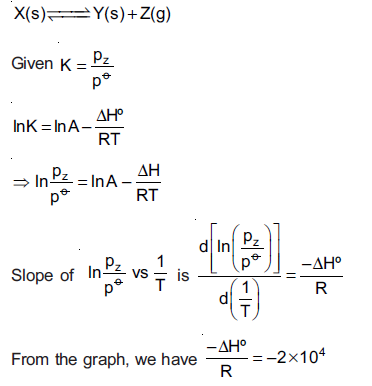⇒ ΔHº = 2 × 104 × 8.314 J

ΔHº = 166.28 kJ mol-1

Question 8. The value of

$$ΔS^{\varnothing}$$
(in J K–1 mol–1) for the given reaction, at 1000 K is ____.

Solution:

–RTln K = ΔGº = ΔHº – TΔSº

Ink = Hº / RT + Sº / R

ΔSº / R = 17

ΔSº = 17R

= 141.338 J K-1

Question Stem for Questions 9 and 10.

The boiling point of water in a 0.1 molal silver nitrate solution (solution A) is x ºC. To this solution A, an equal volume of 0.1 molal aqueous barium chloride solution is added to make a new solution B. The difference in the boiling points of water in the two solutions A and B is y × 10-2 ºC.

(Assume: Densities of the solutions A and B are the same as that of water and the soluble salts dissociate completely. Use: Molal elevation constant (Ebullioscopic constant), Kb = 0.5 K kg mol-1; Boiling point of pure water as 100ºC.)

Question 9. The value of x is ______.

Solution:

Question 10. The value of |y| is ______.

Solution:

Given molality of AgNO3 solution is 0.1 molal (solution-A)

ΔTb = ikb m

AgNO3 → Ag+ + NO3 -

van't Hoff factor (i) for AgNO3 = 2

ΔTb = 2 × 0.5 × 0.1

(Ts – Tº) = 0.1

(Ts)A = 100.1ºC, so x = 100.1

Now solution - A of equal volume is mixed with 0.1 molal BaCl2 solution to get solution-B. AgNO3 reacts with BaCl2 to form AgCl(s).

0.1 mole of AgNO3 present in 1000 gram solvent or 1017 gram or 1017 mL solution,

milli moles of AgNO3 in V ml 0.1 molal solution is nearly 0.1 V. Similarly in BaCl2.

2AgNO3(aq) + BaCl2(aq) → 2AgCl(s) + Ba(NO3)2 (aq)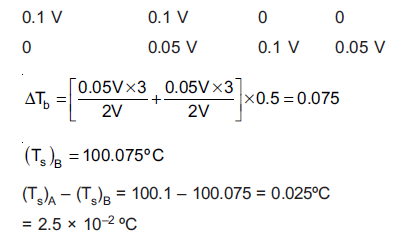So x = 100.1 and |y| = 2.5

Question 11. Given: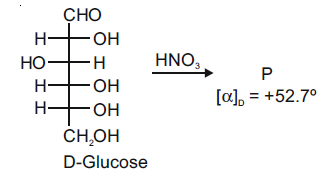The compound(s), which on reaction with HNO3 will give the product having a degree of rotation, [α]D = –52.7º is(are);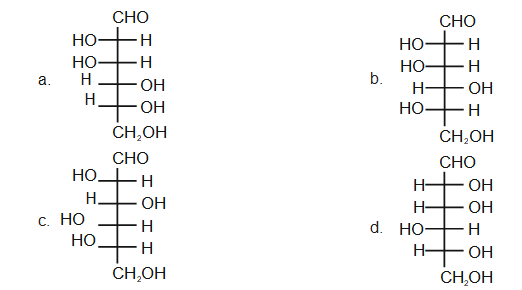Solution: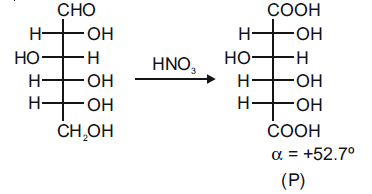The enantiomer of (P) will have –52.7º rotation. So the reactant must be an isomer of D-glucose which can given the mirror image of (P)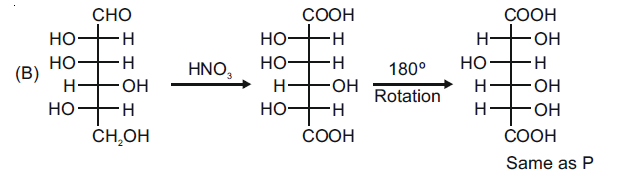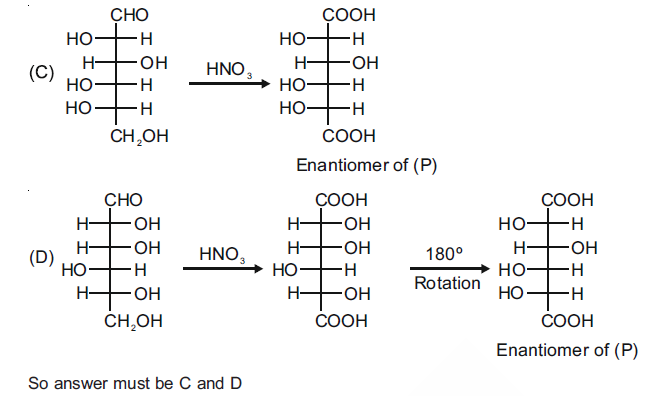Question 12. The reaction of Q with PhSNa yields an organic compound (major product) that gives a positive Carius test on treatment with Na2O2 followed by the addition of BaCl2. The correct option(s) for Q is(are).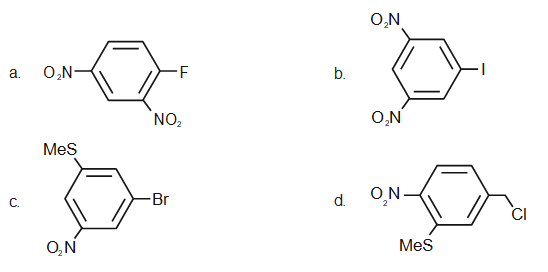Solution: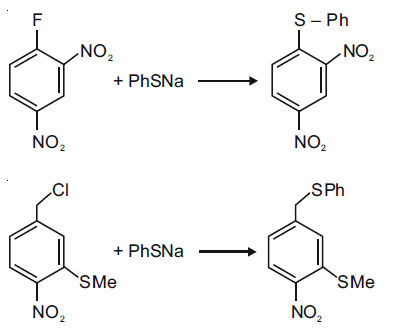The answer should be (a) and (d)

Compounds given in options - b and c do not react with PhSNa.

Question 13. The correct statement(s) related to colloids is(are)

1. a. The process of precipitating colloidal sol by an electrolyte is called peptization
2. b. Colloidal solution freezes at a higher temperature than the true solution at the same concentration
3. c. Surfactants form micelle above critical micelle concentration (CMC). CMC depends on temperature
4. d. Micelles are macromolecular colloids

Solution:

(a) The process of precipitating colloidal sol by an electrolyte is called peptization - False, (It is a process of converting precipitate into colloid)

(b) Colloidal solution freezes at a higher temperature than the true solution at the same concentration - True (colligative properties)

(c) Surfactants form micelles above critical micelle concentration (CMC). CMC depends on temperature - True

(d) Micelles are macromolecular colloids - False, As micelles are associated colloids.

Question 14. An ideal gas undergoes a reversible isothermal expansion from the state I to state II followed by a reversible adiabatic expansion from state II to state III. The correct plot(s) representing the changes from the state I to state III is(are) (p: pressure, V: volume, T: temperature, H: enthalpy, S: entropy)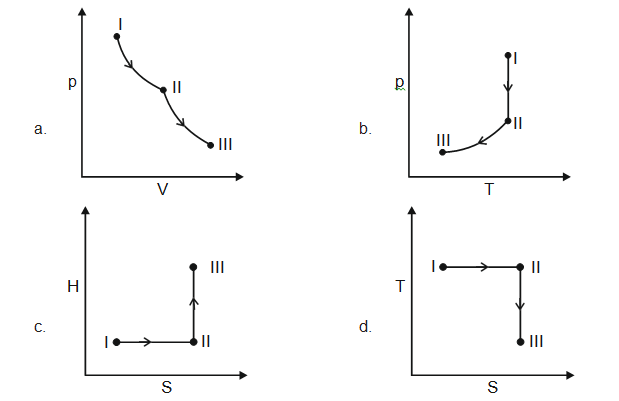Solution:

I → II → reversible, isothermal expansion,

T → constant, ΔV → +ve, ΔS → +ve ΔH ⇒ 0

II → III → Reversible, adiabatic expansion

Q = 0, ΔV → +ve, ΔS → 0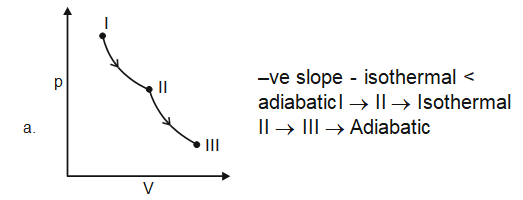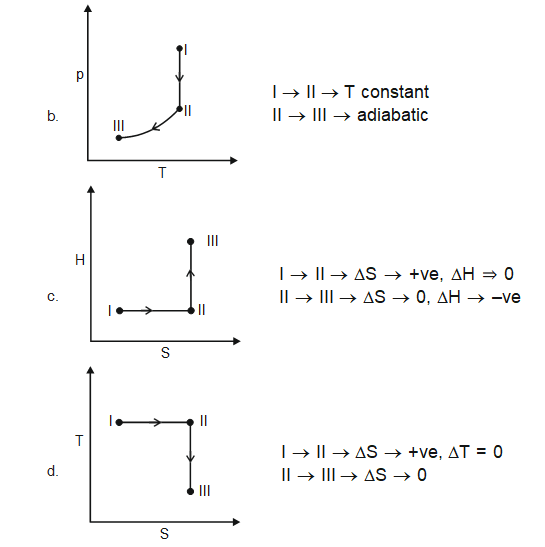Question 15. The correct statement(s) related to the metal extraction processes is(are);

1. a. A mixture of PbS and PbO undergoes self-reduction to produce Pb and SO2.
2. b. In the extraction process of copper from copper pyrites, silica is added to produce copper silicate.
3. c. Partial oxidation of sulphide ore of copper by roasting, followed by self-reduction produces blister copper.
4. d. In the cyanide process, zinc powder is utilized to precipitate gold from Na[Au(CN)2].

Solution:

PbS + 2PbO →3Pb + SO2

Self-reduction is taking place between PbS and PbO.

In the Bessemer converter: The raw material for the Bessemer converter is matte, i.e., Cu2S + FeS (little). Here air blasting is initially done for slag formation and SiO2 is added from an external source.

FeS + 3/2 O2 → FeO + SO2

SiO2 + FeO → FeSiO3 (slag)

During slag formation, the characteristic green flame is observed at the mouth of the Bessemer converter which indicates the presence of iron in the form of FeO. The disappearance of this green flame indicates that the slag formation is complete. Then air blasting is stopped and slag is removed.

Again air blasting is restarted for partial roasting before self-reduction until two-thirds of Cu2S is converted into Cu2O. After this, only heating is continued for the self-reduction process.

Cu2S + 3/2 O2 → Cu2O + SO2

Cu2S + 2Cu2O → 6Cu(l) + SO2 ↑ (self reduction)

and

Cu2S + 2O2 →Cu2SO4

Cu2S + Cu2SO4 → 4Cu + 2SO2 ↑ (self reduction)

Thus the molten Cu obtained is poured into a large container and allowed to cool and during cooling the dissolved SO2 comes up to the surface and forms blisters. It is known as blister copper.

2Na[Au(CN)2 ] + Zn → Na2[Zn(CN)4] + 2Au ↓

Question 16. A mixture of two salts is used to prepare a solution S, which gives the following results: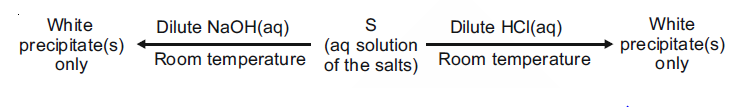The correct option(s) for the salt mixture is(are)

1. a. Pb(NO3)2 and Zn(NO3)2
2. b. Pb(NO3)2 and Bi(NO3)2
3. c. AgNO3 and Bi(NO3)3
4. d. Pb(NO3)2 and Hg(NO3)2

Solution: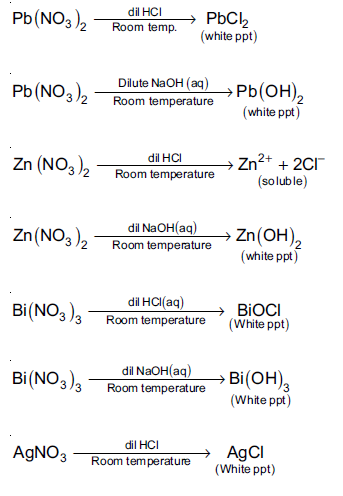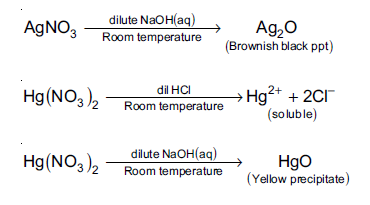Question 17. The maximum number of possible isomers (including stereoisomers) which may be formed on mono-bromination of 1-methylcyclohex-1-ene using Br2 and UV light is ______.

Solution: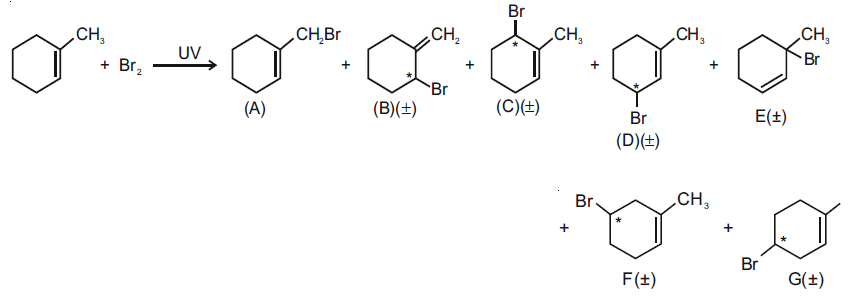Monobromination of 1-methylcyclohexene in the presence of UV light proceeds by a free radical mechanism. The allyl radicals are formed which are stabilised by resonance. The secondary alkyl radicals are also formed which are stabilised by hyperconjugation. Of the seven products formed, six of them are optically active. So, 13 possible isomers are formed.

Question 18. In the reaction given below, the total number of atoms having sp2 hybridization in the major product P is ______.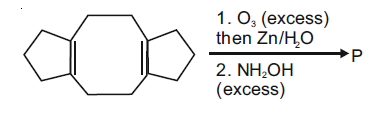Solution: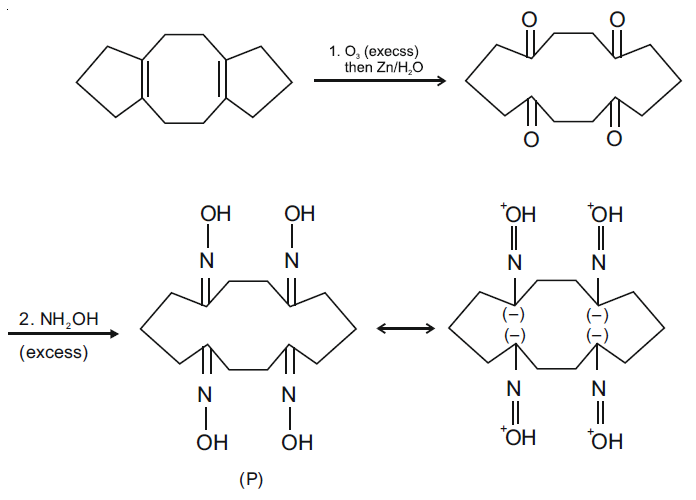The total number of atoms having sp2 hybridisation in the major product (P) = 12

This includes 4 C-atoms, 4 N-atoms and 4 O-atoms.

Question 19. The total number of possible isomers for [Pt(NH3)4Cl2]Br2 is

Solution:

The given complex [Pt(NH3)4Cl2]Br2 has three ionisation isomers and each of them has two geometrical isomers.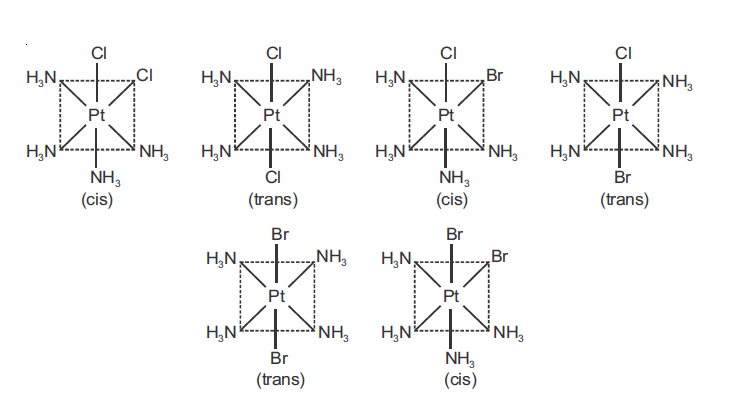### JEE Advanced 2021 Chemistry Paper 1 Solutions